Courses

# Olympiad Test: Mirror Images -2

## 10 Questions MCQ Test Science Olympiad Class 6 | Olympiad Test: Mirror Images -2

Description
This mock test of Olympiad Test: Mirror Images -2 for Class 6 helps you for every Class 6 entrance exam. This contains 10 Multiple Choice Questions for Class 6 Olympiad Test: Mirror Images -2 (mcq) to study with solutions a complete question bank. The solved questions answers in this Olympiad Test: Mirror Images -2 quiz give you a good mix of easy questions and tough questions. Class 6 students definitely take this Olympiad Test: Mirror Images -2 exercise for a better result in the exam. You can find other Olympiad Test: Mirror Images -2 extra questions, long questions & short questions for Class 6 on EduRev as well by searching above.
QUESTION: 1

Solution:
QUESTION: 2

Solution:
QUESTION: 3

### Directions: In each of the following questions, choose the correct mirror image of the figure (X) from amongst the four alternatives (a), (b), (c) and (d) given along with Fig. (X).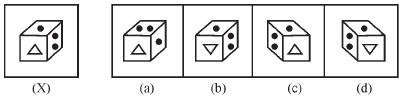Solution:
QUESTION: 4

Directions: In each of the following questions, choose the correct mirror image of the figure (X) from amongst the four alternatives (a), (b), (c) and (d) given along with Fig. (X).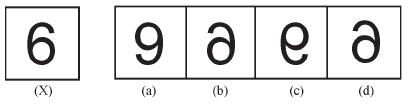Solution:
QUESTION: 5

Directions: In each of the following questions, choose the correct mirror image of the figure (X) from amongst the four alternatives (a), (b), (c) and (d) given along with Fig. (X).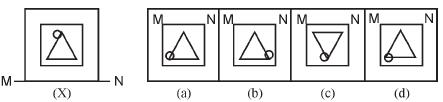Solution:
QUESTION: 6

Directions: In each of the following questions, choose the correct mirror image of the figure (X) from amongst the four alternatives (a), (b), (c) and (d) given along with Fig. (X).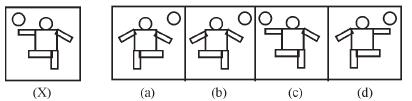Solution:
QUESTION: 7

Directions: In each of the following questions, choose the correct mirror image of the figure (X) from amongst the four alternatives (a), (b), (c) and (d) given along with Fig. (X).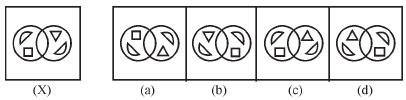Solution:
QUESTION: 8

Directions: In each of the following questions, choose the correct mirror image of the figure (X) from amongst the four alternatives (a), (b), (c) and (d) given along with Fig. (X).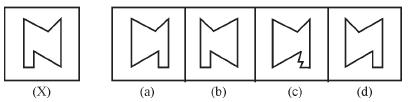Solution:
QUESTION: 9

Directions: In each of the following questions, choose the correct mirror image of the figure (X) from amongst the four alternatives (a), (b), (c) and (d) given along with Fig. (X).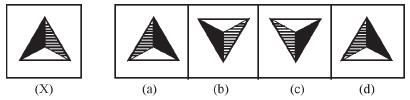Solution:
QUESTION: 10

Directions: In each of the following questions, choose the correct mirror image of the figure (X) from amongst the four alternatives (a), (b), (c) and (d) given along with Fig. (X).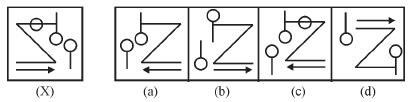Solution: